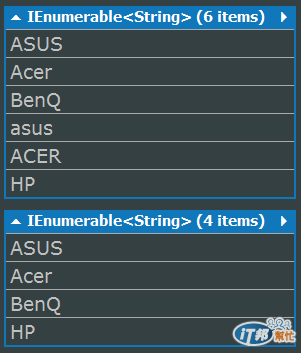#DAY 26
1

## LINQ自學筆記-語法應用-設定方法-Range、Repeat、Empty、Distinct

Range 運算子可以讓我們產生指定範圍內的「整數」序列：

``````public static IEnumerable<int> Range(
int start,
int count
)
``````

``````var squares = Enumerable.Range(2, 4).Select(i => i * i);
foreach (var num in squares)
{
Console.WriteLine(num);
}
Console.WriteLine(squares.GetType());

/* 輸出：
4
9
16
25
typeof (IEnumerable<Int32>)
*/
``````

Repeat 運算子可以讓我們產生一個序列，包含指定數量的元素（或稱之為項目），其方法簽名碼如下：

``````public static IEnumerable<TResult> Repeat<TResult>(
TResult element,
int count
)
``````

``````var echoes = Enumerable.Repeat("Hello~ ", 3);
foreach (var s in echoes)
{
Console.WriteLine(s);
}
Console.WriteLine(echoes.GetType());
/* 輸出：
Hello~
Hello~
Hello~
typeof (IEnumerable<String>)
*/
``````

Empty 運算子可以讓我們建立一個包含指定型別的空序列：

``````public static IEnumerable<TResult> Empty<TResult>()
``````

Empty 運算子也是 Enumerable 的靜態方法，非擴充方法。呼叫時，不用傳入任何參數，只要告訴它要建立序列所要收容的型別就行了：

``````IEnumerable<Decimal> pays = Enumerable.Empty<Decimal>();
Console.WriteLine(pays.GetType());
// 輸出：typeof (Decimal[])
``````

``````public static IEnumerable<TSource> DefaultIfEmpty<TSource>(
this IEnumerable<TSource> source
)
``````

DefaultIfEmpty 運算子是 IEnumerable<TSource> 的擴充方法，不須傳入任何參數，在來源序列上呼叫時，若來源序列是空的，則會回傳 TSource 型別預設值：

``````IEnumerable<Decimal> pays = Enumerable.Empty<Decimal>();
foreach (var e in pays)
{
Console.WriteLine(e);
}
Console.WriteLine("----------------");
Console.WriteLine(pays.DefaultIfEmpty());
/* 輸出：
----------------
0
*/
``````

PS. 若來源序最是 null，執行時會發生 ArgumentNullException 例外。

``````public static IEnumerable<TSource> Distinct<TSource>(
this IEnumerable<TSource> source
)
public static IEnumerable<TSource> Distinct<TSource>(
this IEnumerable<TSource> source,
IEqualityComparer<TSource> comparer
)
``````

``````void Main()
{
var list = new List<string>() {"ASUS","Acer","BenQ", "asus", "ACER", "HP"};
var comparer = new IgnoreCaseSensitive();
list.Distinct().Dump();
list.Distinct(comparer).Dump();
}
//自訂忽略大小寫的相等比較子
public class IgnoreCaseSensitive : IEqualityComparer<string>
{
public bool Equals(string s1, string s2)
{
return (s1.ToUpper().Equals(s2.ToUpper()));
}
public int GetHashCode(string str)
{
return str.ToUpper().GetHashCode();
}
}
``````